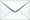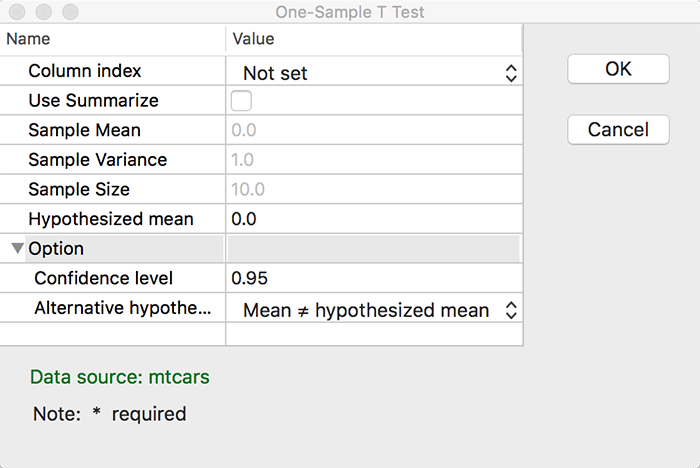Product Search

Dear customers:

take action as soon as possible.tyevolution@yahoo.com

# Analyze: One-Sample T Test

Views:355timesColumn Index: Choose a numeric vector of data values in columns.

Use Summarize: Choose if you have summary values for the sample size, mean, and varience.

Sample Mean: Enter the value for the sample mean.

Sample Varience: Enter the value for the sample varience. It is a numeric constant. Value > 0

Sample Size: Enter the value for the sample size. It is a integer constant. Value > 1

Hypothesized Mean: Enter the test mean.

Option
Confidence Level: Enter the level of confidence desired. Enter any number between 0 and 1.

Alternative Hypothesis: It contains Mean ≠ Hypothesized Mean (two-tailed),

Mean < Hypothesized Mean (lower-tailed),

Mean > Hypothesized Mean (upper-tailed).

If you choose a lower-tailed or an upper-tailedhypothesis test,

an upper or lower confidence bound will be constructed, respectively,

rather than a confidence interval.